The non-Markovian nature of turbulence 8: Almost-Markovian models and theories

Previously, in my post of 10 November 2022, I mentioned, purely for completeness, the work of Phythian  who presented a self-consistent theory that led to the DIA. The importance of this for Kraichnan was that it also led to a model representation of the DIA and in turn to the development of what he called almost-Markovian’ theories. Some further discussion of this topic can be found in Section 6.3.2 of the book , but here we will concentrate on the general class of almost-Markovian models and theories. My concern here is to draw a distinction between their use of Markovian’, which refers to evolution in time, and my use in this series of posts, which refers to interactions in wavenumber.

This class consists of the Eddy-damped, Quasi-normal, Markovian (EDQNM) model of Orszag in 1970 , the test-field model of Kraichnan in 1971 , the modified LET theory of McComb and Kiyani in 2005 , and the theory of Bos and Bertoglio in 2006 . Here we follow the example of Kraichnan who described a theory which relied on a specific assumption that involved the introduction of an adjustable constant as a model. In order to illustrate what is going on in this kind of approach, I will discuss the EDQNM in some detail, as follows.

We begin with the quasi-normal expression for the transfer spectrum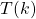from the Lin equation. This is found to be:

(1)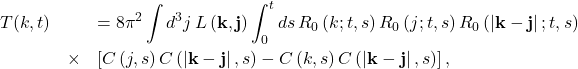where the viscous response function is given by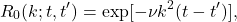and the coefficient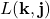is defined as:

(2)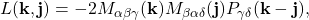and can be evaluated in terms of three scalar variables as

(3)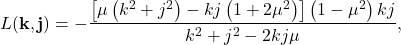whereis the cosine of the angle between the vectors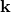and. For further discussion and details see Appendix C of the book .

Now Orszag argued that the failure of QN was basically due to the use of the viscous response function, when in fact one would expect that the turbulence interactions would contribute to the response function. Accordingly he proposed a modified response function:

(4)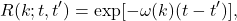where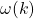is a renormalized inverse modal response time. One may note that this is now becoming the same form as that of the Edwards transfer spectrum, but that it is also ad hoc and thus there is the freedom to choose. After some experimentation using dimensional analysis, Orszag chose the form:

(5)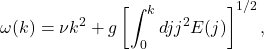where the constant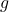is chosen to give the correct (i.e. experimental) result for the Kolmogorov spectrum. This is the eddy damped part of the model, so replacing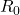by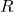gives us the EDQN.

Even with the introduction of the damping term, the EDQN model can still lead to negative spectra. This was cured by introducing the Markovian step with respect to time. This rested on the assumption that the characteristic time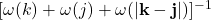is negligible compared to the evolution time of the products of covariances in the expression for. The equation for the transfer spectrum was Markovianised by replacing the time integral by a memory function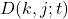, thus:

(6)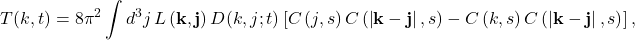where the memory function is given by

(7)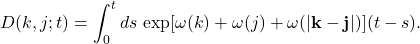This is now the EDQNM model.

When applied to the stationary case, this result foris identical to the Edwards result, as given in the post of 3 November 2022; but there are crucial differences. The functionin the Edwards theory arises from a Markovian theory with respect to wavenumber interactions and is accordingly related to, thus giving the second equation of the closure. In contrast, the functionin EDQNM is fixed independently of the transfer spectrum by means of dimensional analysis and accordingly is not Markovian in the sense of the Edwards SCF. It is important to distinguish between the two kinds of Markovianisation.

In our next post, we will conclude this series of posts by discussing how these considerations affect the application of closures to large-eddy simulation.

 R. Phythian. Self-consistent perturbation series for stationary homogeneous turbulence. J.Phys.A, 2:181, 1969.
 W. D. McComb. The Physics of Fluid Turbulence. Oxford University Press, 1990.
 S. A. Orszag. Analytical theories of turbulence. J. Fluid Mech., 41:363, 1970.
 R. H. Kraichnan. An almost-Markovian Galilean-invariant turbulence model. J. Fluid Mech., 47:513, 1971.
 W. D. McComb and K. Kiyani. Eulerian spectral closures for isotropic turbulence using a time-ordered fluctuation-dissipation relation. Phys. Rev. E, 72:16309{16312, 2005.
 W. J. T. Bos and J.-P. Bertoglio. A single-time, two-point closure based on fluid particle displacements. Phys. Fluids, 18:031706, 2006.
 W. David McComb. Homogeneous, Isotropic Turbulence: Phenomenology, Renormalization and Statistical Closures. Oxford University Press, 2014.